## The Relationship Between Present and Future Value

Present value (PV) and future value (FV) measure how much the value of money has changed over time.

### Learning Objectives

Discuss the relationship between present value and future value

### Key Takeaways

#### Key Points

• The future value (FV) measures the nominal future sum of money that a given sum of money is “worth” at a specified time in the future assuming a certain interest rate, or more generally, rate of return. The FV is calculated by multiplying the present value by the accumulation function.
• PV and FV vary jointly: when one increases, the other increases, assuming that the interest rate and number of periods remain constant.
• As the interest rate ( discount rate) and number of periods increase, FV increases or PV decreases.

#### Key Terms

• discounting: The process of finding the present value using the discount rate.
• present value: a future amount of money that has been discounted to reflect its current value, as if it existed today
• capitalization: The process of finding the future value of a sum by evaluating the present value.

The future value (FV) measures the nominal future sum of money that a given sum of money is “worth” at a specified time in the future assuming a certain interest rate, or more generally, rate of return. The FV is calculated by multiplying the present value by the accumulation function. The value does not include corrections for inflation or other factors that affect the true value of money in the future. The process of finding the FV is often called capitalization.

On the other hand, the present value (PV) is the value on a given date of a payment or series of payments made at other times. The process of finding the PV from the FV is called discounting .

PV and FV are related, which reflects compounding interest ( simple interest has n multiplied by i, instead of as the exponent). Since it’s really rare to use simple interest, this formula is the important one.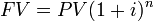FV of a single payment: The PV and FV are directly related.

PV and FV vary directly: when one increases, the other increases, assuming that the interest rate and number of periods remain constant.

The interest rate (or discount rate) and the number of periods are the two other variables that affect the FV and PV. The higher the interest rate, the lower the PV and the higher the FV. The same relationships apply for the number of periods. The more time that passes, or the more interest accrued per period, the higher the FV will be if the PV is constant, and vice versa.

The formula implicitly assumes that there is only a single payment. If there are multiple payments, the PV is the sum of the present values of each payment and the FV is the sum of the future values of each payment.

## Calculating Perpetuities

The present value of a perpetuity is simply the payment size divided by the interest rate and there is no future value.

### Learning Objectives

Calculate the present value of a perpetuity

### Key Takeaways

#### Key Points

• Perpetuities are a special type of annuity; a perpetuity is an annuity that has no end, or a stream of cash payments that continues forever.
• To find the future value of a perpetuity requires having a future date, which effectively converts the perpetuity to an ordinary annuity until that point.
• Perpetuities with growing payments are called Growing Perpetuities; the growth rate is subtracted from the interest rate in the present value equation.

#### Key Terms

• growth rate: The percentage by which the payments grow each period.

Perpetuities are a special type of annuity; a perpetuity is an annuity that has no end, or a stream of cash payments that continues forever. Essentially, they are ordinary annuities, but have no end date. There aren’t many actual perpetuities, but the United Kingdom has issued them in the past.

Since there is no end date, the annuity formulas we have explored don’t apply here. There is no end date, so there is no future value formula. To find the FV of a perpetuity would require setting a number of periods which would mean that the perpetuity up to that point can be treated as an ordinary annuity.

There is, however, a PV formula for perpetuities. The PV is simply the payment size (A) divided by the interest rate (r). Notice that there is no n, or number of periods. More accurately, is what results when you take the limit of the ordinary annuity PV formula as n → ∞.

It is also possible that an annuity has payments that grow at a certain rate per period. The rate at which the payments change is fittingly called the growth rate (g). The PV of a growing perpetuity is represented as $\text{PVGP} \ = \ {\text{A} \over ( \text{i} - \text{g} )}$. It is essentially the same as in except that the growth rate is subtracted from the interest rate. Another way to think about it is that for a normal perpetuity, the growth rate is just 0, so the formula boils down to the payment size divided by r.

## Calculating Values for Different Durations of Compounding Periods

Finding the Effective Annual Rate (EAR) accounts for compounding during the year, and is easily adjusted to different period durations.

### Learning Objectives

Calculate the present and future value of something that has different compounding periods

### Key Takeaways

#### Key Points

• The units of the period (e.g. one year) must be the same as the units in the interest rate (e.g. 7% per year).
• When interest compounds more than once a year, the effective interest rate (EAR) is different from the nominal interest rate.
• The equation in skips the step of solving for EAR, and is directly usable to find the present or future value of a sum.

#### Key Terms

• present value: Also known as present discounted value, is the value on a given date of a payment or series of payments made at other times. If the payments are in the future, they are discounted to reflect the time value of money and other factors such as investment risk. If they are in the past, their value is correspondingly enhanced to reflect that those payments have been (or could have been) earning interest in the intervening time. Present value calculations are widely used in business and economics to provide a means to compare cash flows at different times on a meaningful “like to like” basis.
• Future Value: The value of an asset at a specific date. It measures the nominal future sum of money that a given sum of money is “worth” at a specified time in the future, assuming a certain interest rate, or more generally, rate of return, it is the present value multiplied by the accumulation function.

Sometimes, the units of the number of periods does not match the units in the interest rate. For example, the interest rate could be 12% compounded monthly, but one period is one year. Since the units have to be consistent to find the PV or FV, you could change one period to one month. But suppose you want to convert the interest rate into an annual rate. Since interest generally compounds, it is not as simple as multiplying 1% by 12 (1% compounded each month). This atom will discuss how to handle different compounding periods.

### Effective Annual Rate

The effective annual rate (EAR) is a measurement of how much interest actually accrues per year if it compounds more than once per year. The EAR can be found through the formula in where i is the nominal interest rate and n is the number of times the interest compounds per year (for continuous compounding, see ). Once the EAR is solved, that becomes the interest rate that is used in any of the capitalization or discounting formulas.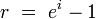EAR with Continuous Compounding: The effective rate when interest compounds continuously.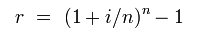Calculating the effective annual rate: The effective annual rate for interest that compounds more than once per year.

For example, if there is 8% interest that compounds quarterly, you plug.08 in for i and 4 in for n. That calculates an EAR of.0824 or 8.24%. You can think of it as 2% interest accruing every quarter, but since the interest compounds, the amount of interest that actually accrues is slightly more than 8%. If you wanted to find the FV of a sum of money, you would have to use 8.24% not 8%.

### Solving for Present and Future Values with Different Compounding Periods

Solving for the EAR and then using that number as the effective interest rate in present and future value (PV/FV) calculations is demonstrated here. Luckily, it’s possible to incorporate compounding periods into the standard time-value of money formula. The equation in is the same as the formulas we have used before, except with different notation. In this equation, A(t) corresponds to FV, A0 corresponds to Present Value, r is the nominal interest rate, n is the number of compounding periods per year, and t is the number of years.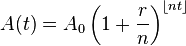FV Periodic Compounding: Finding the FV (A(t)) given the PV (Ao), nominal interest rate (r), number of compounding periods per year (n), and number of years (t).

The equation follows the same logic as the standard formula. r/n is simply the nominal interest per compounding period, and nt represents the total number of compounding periods.

### Solving for n

The last tricky part of using these formulas is figuring out how many periods there are. If PV, FV, and the interest rate are known, solving for the number of periods can be tricky because n is in the exponent. It makes solving for n manually messy. shows an easy way to solve for n. Remember that the units are important: the units on n must be consistent with the units of the interest rate (i).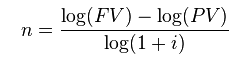Solving for n: This formula allows you to figure out how many periods are needed to achieve a certain future value, given a present value and an interest rate.

## Comparing Interest Rates

Variables, such as compounding, inflation, and the cost of capital must be considered before comparing interest rates.

### Learning Objectives

Discuss the differences between effective interest rates, real interest rates, and cost of capital

### Key Takeaways

#### Key Points

• A nominal interest rate that compounds has a different effective rate (EAR), because interest is accrued on interest.
• The Fisher Equation approximates the amount of interest accrued after accounting for inflation.
• A company will theoretically only invest if the expected return is higher than their cost of capital, even if the return has a high nominal value.

#### Key Terms

• inflation: An increase in the general level of prices or in the cost of living.

The amount of interest you would have to pay on a loan or would earn on an investment is clearly an important consideration when making any financial decisions. However, it is not enough to simply compare the nominal values of two interest rates to see which is higher.

### Effective Interest Rates

The reason why the nominal interest rate is only part of the story is due to compounding. Since interest compounds, the amount of interest actually accrued may be different than the nominal amount. The last section went through one method for finding the amount of interest that actually accrues: the Effective Annual Rate (EAR).

The EAR is a calculation that account for interest that compounds more than one time per year. It provides an annual interest rate that accounts for compounded interest during the year. If two investments are otherwise identical, you would naturally pick the one with the higher EAR, even if the nominal rate is lower.

### Real Interest Rates

Interest rates are charged for a number of reasons, but one is to ensure that the creditor lowers his or her exposure to inflation. Inflation causes a nominal amount of money in the present to have less purchasing power in the future. Expected inflation rates are an integral part of determining whether or not an interest rate is high enough for the creditor.

The Fisher Equation is a simple way of determining the real interest rate, or the interest rate accrued after accounting for inflation. To find the real interest rate, simply subtract the expected inflation rate from the nominal interest rate.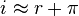Fisher Equation: The nominal interest rate is approximately the sum of the real interest rate and inflation.

For example, suppose you have the option of choosing to invest in two companies. Company 1 will pay you 5% per year, but is in a country with an expected inflation rate of 4% per year. Company 2 will only pay 3% per year, but is in a country with an expected inflation of 1% per year. By the Fisher Equation, the real interest rates are 1% and 2% for Company 1 and Company 2, respectively. Thus, Company 2 is the better investment, even though Company 1 pays a higher nominal interest rate.

### Cost of Capital

Another major consideration is whether or not the interest rate is higher than your cost of capital. The cost of capital is the rate of return that capital could be expected to earn in an alternative investment of equivalent risk. Many companies have a standard cost of capital that they use to determine whether or not an investment is worthwhile.

In theory, a company will never make an investment if the expected return on the investment is less than their cost of capital. Even if a 10% annual return sounds really nice, a company with a 13% cost of capital will not make that investment.

## Calculating Values for Fractional Time Periods

The value of money and the balance of the account may be different when considering fractional time periods.

### Learning Objectives

Calculate the future and present value of an account when a fraction of a compounding period has passed

### Key Takeaways

#### Key Points

• The balance of an account only changes when interest is paid. To find the balance, round the fractional time period down to the period when interest was last accrued.
• To find the PV or FV, ignore when interest was last paid an use the fractional time period as the time period in the equation.
• The discount rate is really the cost of not having the money over time, so for PV/FV calculations, it doesn’t matter if the interest hasn’t been added to the account yet.

#### Key Terms

• time period assumption: business profit or loses are measured on timely basis
• compounding period: The length of time between the points at which interest is paid.
• time value of money: the value of an asset accounting for a given amount of interest earned or inflation accrued over a given period

Up to this point, we have implicitly assumed that the number of periods in question matches to a multiple of the compounding period. That means that the point in the future is also a point where interest accrues. But what happens if we are dealing with fractional time periods?

Compounding periods can be any length of time, and the length of the period affects the rate at which interest accrues.### Principle and Interest

As a result of this calculation, amortization schedules charge interest over time as a percentage of the principal borrowed. The calculation will incorporate the number of payment periods (n), the principal (P), the amortization payment (A) and the interest rate (r).

$\text{P}\,=\,\text{A}\cdot {\frac {1-\left({\frac {1}{1+\text{r}}}\right)^{\text{n}}}{\text{r}}}$

To make this a bit more realistic, let’s insert some numbers. Let’s say you find a dream house, at the reasonable rate of $100,000. Unfortunately, a bit of irresponsible borrowing in your past means you must pay 8% interest over a 30 year loan, which will be paid via a monthly amortization schedule (12 months x 30 years = 360 payments total). If you do the math, you should find yourself paying$734 per month 360 times. 360 x 734 will leave you in the ballpark of $264,000 in total repayment. that means you are paying more than 2.5 times as much for this house due to time value of money! This bit of knowledge is absolutely critical for personal financial decisions, as well as for high level business decisions.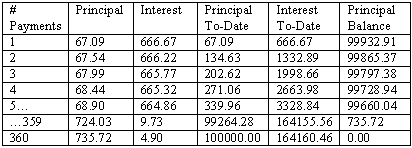Amortization Schedule Example: This shows the first few installments in the example discussed above (i.e. borrowing$100,000 at 8% interest paid monthly over 30 years).